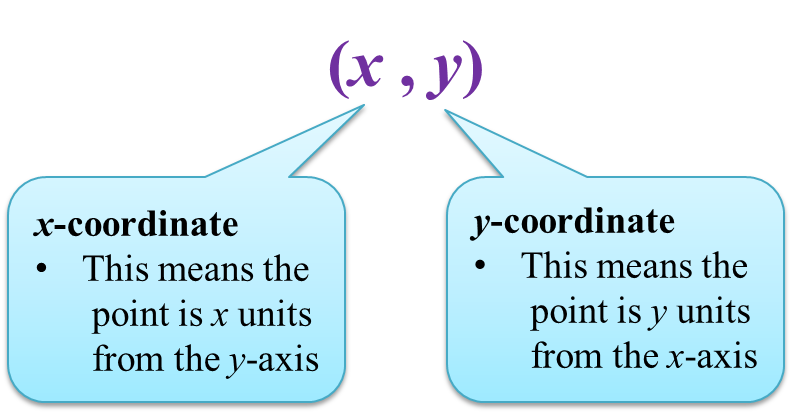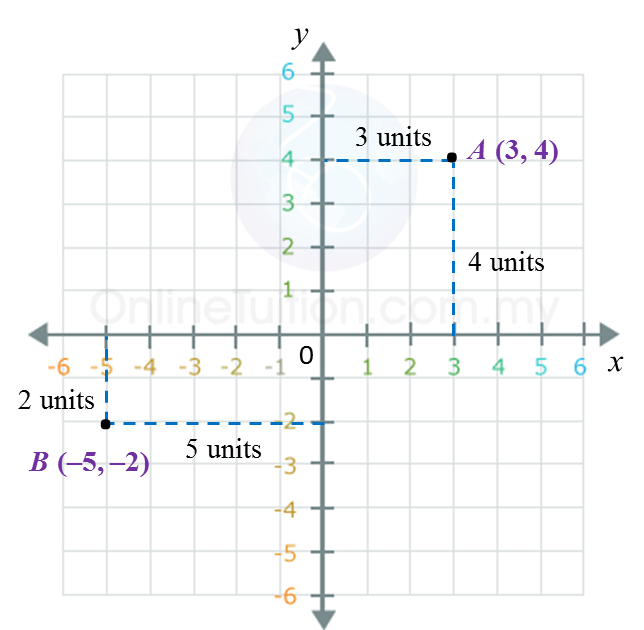# 7.1 Coordinates

7.1 Coordinates

7.1.1 Coordinates
1. The Cartesian coordinate system is a number plane with a horizontal line (x-axis) drawn at right angles to a vertical line (y-axis), intersecting at a point called origin.2. It is used to locate the position of a point in reference to the x-axis and y-axis.

3. The coordinate of any point are written as an ordered pair (x, y).  The first number is the x-coordinate and the second number is the y-coordinate of the point.Example:The coordinates of points A and B are (3, 4) and (–5, –2) respectively.
This means that point A is located 3 units from they-axis and 4 units from the x-axis, whereas point B is located 5 units on the left from the y-axis and 2 units from the x-axis.

4. The coordinate of the origin O is (0, 0).